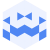# Lynn Mayya | Tresor Linz I October 24 2023 | BestOFF Inside OutCreated at 13. Nov. 2023

113 Ansichten
##### by Tresor Linz

Tresor Linz - recorded on October 24 2023

<<<<<<<<<<<<<<<<<<<<<<<<<<<<<<<<<<<<<<<<<<<<<<<<<<<<<<<<<
LYNN MAYYA - Axis 101
<<<<<<<<<<<<<<<<<<<<<<<<<<<<<<<<<<<<<<<<<<<<<<<<<<<<<<<<<
(Time-based and Interactive Media Arts)

de:
Eine experimentelle, audiovisuelle, interaktive Performance, die Ton und Bild zu einem hypnotisierenden Erlebnis verbindet. Die Bilder basieren auf den Grundfarben RGB, während der Sound glitchige und dröhnende Klänge enthält, die die Bilder in Echtzeit modulieren und beeinflussen. Die Performance lädt die Betrachter*innen ein, sich mit ihren eigenen Wahrnehmungen von Bild und Ton auseinanderzusetzen und die tieferen Verbindungen zwischen beiden zu erforschen.

en:
An experimental audiovisual interactive performance that embodies sound & images to create a hypnotizing experience. The visuals are based on the basic colours, RGB while the sound incorporates glitchy and dronelike sounds that modulate and affect the visuals in real time. This invites viewers to engage with their own perceptions of sound and image, and to explore the deeper connections between both.

<<<<<<<<<<<<<<<<<<<<<<<<<<<<<<<<<<<<<<<<<<<<<<<<<<<<<<<<<
Tresor Linz
<<<<<<<<<<<<<<<<<<<<<<<<<<<<<<<<<<<<<<<<<<<<<<<<<<<<<<<<<

Raum für Klangkunst und künstlerische Experimente.

Space for sound art and artistic experiments.

##### Share & Embed
Embed this Video